## Aug 20, 2009

1. Choose an odd number from the following:

a. 16

b. 12

c. 19

d. 14

2. Which of the following is greater in value?

a. 65

b. 74

c. 45

d. 59

3. The ordinal name of 6 is ____

a. Seventh

b. Sixth

c. Fifth

d. Fourth

4. 5 tens+7 ones=________

a. 57

b. 75

c. 507

d. 705

5. 7+3=_________

a. 5+5

b. 6+3

c. 2+9

d. 8+1

6. There are 14 roses in the garden. 8 of the roses are red and the remaining roses are white. Find the number of white roses in the garden.

a. 5

b. 6

c. 7

d. 8

7. Fill in the sequence:

5, 10, 15, 20, ___, 30, 35.

a. 23

b. 24

c. 25

d. 26

8. 18=18+___

a. 3

b. 2

c. 1

d. 0

9. Fill in the place holder:

37 20=17

a. +

b.

c. =

d. 0

10. Fill in the blank:

8+7=___+8

a. 6

b. 7

c. 8

d. 9

11. The given table shows the list of pet animals maintained in a locality. Which pet is least in number?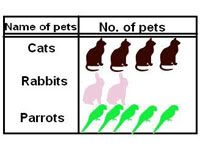a. Cats

b. Rabbits

c. Parrots

d. None of the above

12. Find the missing pattern.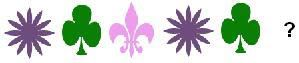a.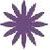b.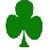c.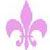d.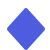13.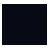The given picture is a ______

a. Square

b. Rectangle

c. Circle

d. Triangle

14. The calendar show today is Tuesday. What day will it be tomorrow?

a. Friday

b. Sunday

c. Wednesday

d. Monday

15. How many months are there in a year?

a. 7

b. 10

c. 12

d. 16

16. Natalie, a first grade student has 5 dollars with her. How does she represent her money value?

a. @ 5

b. % 5

c. # 5

d. \$ 5

17. 1+2+3=___

a. 4

b. 5

c. 6

d. 7

18. Which of following is more than 10?

a. 7

b. 9

c. 10

d. 12

19. Jenny got 5 candies from White, 6 from Pete and 4 from Margaret. Who gave more number of candies to Jenny?

a. White

b. Jenny

c. Pete

d. Margaret

20. What is 1 less than 10?

a. 7

b. 8

c. 9

d. 10

Questions: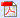Answers: